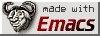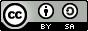# Word list

Funções em elisp para manipulação de ficheiros de palavras.

Na antiga versão do jogo do monopólio existia uma carta da sorte que começava com a expressão "Levou um tiro de um amigo..." e normalmente o que se seguia consistia no pagamento de uma conta do hospital.

Um amigo não me deu um tiro mas fez-me uma pergunta, e no meu caso a procura da resposta leva a quase uma visita ao hospital. A pergunta era: " Será que existem palavras de 5 letras em português que verifiquem o padrão seguinte?"

```1 5 9 1 7
2 6 0 5 6
3 7 3 4 0
4 8 2 8 9
```
Nota: cada número representa uma letra diferente.

Não interessa muito de onde aparece a motivação da pergunta, interessa-me mais a procura da resposta. Há largos meses que tinha começado a ler o volume 4 do TAOCP Combinatorial Algorithms, Part 1 onde, entre outras coisas, é usada a Stanford GraphBase, uma lista de 5757 palavras em inglês com cinco letras, para exposição dos vários algoritmos que aí são descritos.

Propus-me então replicar1 alguns dos resultados descritos no TAOCP usando palavras em PT. Para isso precisava de um dicionário e uma maneira de começar a extrair a informação. O resultado é este pequeno conjunto de funções em elisp. Muito ainda está por fazer, por exemplo, o aspell usa um sistema de compressão para prefixos e sufixos, cuja sintaxe só descobri hoje, e que aumenta o número de palavras disponíveis para se brincar.

```;;; wlist.el --- Emacs tools for manipulating word-files
;; word-files, meaning a file with words in it, one word per line.

;; Author: tca
;; Maintainer: tca
;; Created: Fev, 17, 2012
;; Version: .1
;; Keywords: words
;; URL: https://nexp.pt/wlist.html

;; Copyright (c) 2012 Tiago Charters de Azevedo

;; This file is not part of GNU Emacs.

;; This program is free software; you can redistribute it and/or modify
;; the Free Software Foundation; either version 3, or (at your option)
;; any later version.

;; This program is distributed in the hope that it will be useful,
;; but WITHOUT ANY WARRANTY; without even the implied warranty of
;; MERCHANTABILITY or FITNESS FOR A PARTICULAR PURPOSE.  See the
;; GNU General Public License for more details.

;; You should have received a copy of the GNU General Public License
;; along with this program; if not, write to the Free Software
;; Foundation, Inc., 51 Franklin Street, Fifth Floor,
;; Boston, MA 02110-1301, USA.

;;; Commentary:
;; None.

;; Code:

(defvar wlist-max-lenght
0
"")

(defvar wlist-regexp-pt
"[aerisontcdmlupvgbfzáhçqjíxãóéêâúõACMPSBTELGRIFVDkHJONôywUKXZWQÁYÍÉàÓèÂÚ].+"
"Regular expression for a portuguese word; see aspell.")

(defun wlist-looking-at-size ()
"Returns the length of a word on the beginning of a line (pt-PT)."
(interactive)
(if  (looking-at wlist-regexp-pt)
(length (match-string-no-properties 0))
0))

(defun  wlist-looking-at-size-plus-1 ()
(interactive)
(forward-line 1)
(wlist-looking-at-size))

(defun wlist (size)
"Removes all sized SIZE words from `current-buffer'; asks SIZE."
(interactive "n")
(with-current-buffer (current-buffer)
(goto-char (point-min))
(save-excursion
(while (< (line-number-at-pos) (line-number-at-pos (point-max)))
(when (not (= size (wlist-looking-at-size)))
(delete-region (point) (line-end-position)))
(forward-line 1))))
(wlist-delete-blank-lines))

(defun wlist-delete-blank-lines ()
(interactive)
(with-current-buffer (current-buffer)
(goto-char (point-min))
(save-excursion
(while (< (line-number-at-pos) (line-number-at-pos (point-max)))
(delete-blank-lines)
(forward-line 1)))))

"Inserts a not so beautiful header."
(goto-char (point-min))
(insert (format "File name: %s\n" (file-name-nondirectory (buffer-file-name)))
(format "Number of %s sized words: %s\n" size (line-number-at-pos (point-max)))))

(defun wlist-hamming-dist-list (lst1 lst2)
"For equal length lists is the number of positions at which the
corresponding lists are different."
(if  (and lst1 lst2
(= (length lst1) (length lst2)))
(if (equal (car lst1) (car lst2))
(wlist-hamming-dist-list (cdr lst1) (cdr lst2))
(+ 1 (wlist-hamming-dist-list (cdr lst1) (cdr lst2))))
0))

(defun wlist-hamming-dist (str1 str2)
"For equal length strings is the number of positions at which the
corresponding strings are different."
(wlist-hamming-dist-list (string-to-list str1)
(string-to-list str2)))

(defun wlist-remove-bslash ()
"Remove /* properties form word-file. No plurals or m/f, etc,...
Needs to be changed correctly for portuguese."
(interactive)
(with-current-buffer (current-buffer)
(goto-char (point-min))
(save-excursion
(while (search-forward-regexp "\\(.+\\)\\(/.+\\)" nil t)
(let ((word-s (match-string-no-properties 1)))
(replace-match word-s))))))

(defun wlist-max-size ()
"Gets the maximum size of all words in a word-file."
(interactive)
(with-current-buffer (current-buffer)
(goto-char (point-min))
(save-excursion
(while (< (line-number-at-pos) (line-number-at-pos (point-max)))
(setq wlist-max-lenght (max (wlist-looking-at-size) (wlist-looking-at-size-plus-1) wlist-max-lenght))))))

(defun wlist-file-size (size)
"Determines and creates a file of all the words with size SIZE."
(interactive "n")
(let ((dic-words (buffer-string)))
(with-temp-buffer
(insert dic-words)
(wlist size)
(append-to-file (point-min) (point-max)
(concat default-directory (format "%s.wl" size))))))

(defun wlist-all-files-sizes ()
"Determines all the words from size 2 to `wlist-max-size'
and save them to separate files *.wl; it takes a few minutes to finish."
(interactive)
(if (= wlist-max-lenght 0)
(wlist-max-size))
(let ((size 2))
(while (<= size wlist-max-lenght )
(wlist-file-size size)
(incf size))))

(defun wlist-same-pos (n)
(interactive "x")
(with-current-buffer (current-buffer)
(goto-char (point-min))
(save-excursion
(while (< (line-number-at-pos) (line-number-at-pos (point-max)))
(looking-at wlist-regexp-pt)
(if (not (equal (nth (- (car n) 1 )
(string-to-list (match-string-no-properties 0)))
(string-to-list (match-string-no-properties 0)))))
;;          (message (match-string-no-properties 0)))
(delete-region (point) (line-end-position)))
(forward-line 1)))
(wlist-delete-blank-lines)))

(defun wlist-file-same-pos (n)
(interactive "x")
(let ((i (car n))
(words (buffer-string)))
(with-temp-buffer
(insert words)
(wlist-same-pos (list i j))
(append-to-file (point-min) (point-max)
(concat default-directory (format "%s_%s.wl" i j))))))

(defun wlist-find-all-hamming-dist-word (word dist)
(with-current-buffer (current-buffer)
(goto-char (point-min))
(save-excursion
(while (< (line-number-at-pos) (line-number-at-pos (point-max)))
(looking-at wlist-regexp-pt)
(if (not (= dist (wlist-hamming-dist word (match-string-no-properties 0))))
(delete-region (point) (line-end-position)))
(forward-line 1)))
(wlist-delete-blank-lines)))

(defvar wlist-buffer-content-list nil
"")

(defun wlist-buffer-length ()
(count-lines (point-min) (point-max)))

(defun wlist-buffer-alist ()
(interactive)
(with-current-buffer (current-buffer)
(goto-char (point-min))
(let ((wl-buffer-list nil))
(save-excursion
(while (< (line-number-at-pos) (line-number-at-pos (point-max)))
(looking-at wlist-regexp-pt)
;;    (push 'new-item accumulator)
(push (match-string-no-properties 0) wl-buffer-list)
(forward-line 1)))
(reverse wl-buffer-list))))

"")

(defun wlist-hamming-dist-word-insert (word dist)
"Looks for the hamming DIST of word WORD, inserts all the words found after WORD."
(with-current-buffer (current-buffer)
(goto-char (point-min))
(save-excursion
(while (< (line-number-at-pos) (line-number-at-pos (point-max)))
(looking-at wlist-regexp-pt)
;; Looks for word in file and save point, for inserting.
(if (equal word (match-string-no-properties 0))
(setq w-point (list (point) (line-end-position))))
(forward-line 1)))
(save-excursion
(while (< (line-number-at-pos) (line-number-at-pos (point-max)))
(looking-at wlist-regexp-pt)
(if (= dist (wlist-hamming-dist word (match-string-no-properties 0)))
(forward-line 1)))
(insert (format " %s" (mapconcat 'concat word-link " ")))))

(defun wlist-hamming-dist-insert-buffer (dist)
"Builds the world link with DIST and inserts the result after every word in buffer."
(let ((wlist-list (wlist-buffer-alist)  ))
(dolist (word wlist-list)
(wlist-hamming-dist-word-insert word dist))))

(defun wlist-insert-after-word (word in-word)
"Insert IN-WORD in `curren-buffer' after WORD."
(with-current-buffer (current-buffer)
(goto-char (point-min))
(save-excursion
(while (< (line-number-at-pos) (line-number-at-pos (point-max)))
(looking-at wlist-regexp-pt)
(if (equal word (match-string-no-properties 0))
(replace-match (format "%s %s" word in-word)))
(forward-line 1)))))

(defun wlist-hamming-dist-word-list (word dist)
"Looks for the words with hamming DIST of word WORD; returns all of the in a list."
(with-current-buffer (current-buffer)
(goto-char (point-min))
(save-excursion
(while (< (line-number-at-pos) (line-number-at-pos (point-max)))
(looking-at wlist-regexp-pt)
(if (= dist (wlist-hamming-dist word (match-string-no-properties 0)))
(forward-line 1))))

"Returns the next link of word-link with hamming DIST of word WORD."
(car (wlist-hamming-dist-word-list word dist)))

"Returns a word-link from WORD whit hamming distance DIST."
(while (and new-word (<= 0 n))
(wlist-one-word-link new-word dist (- n 1)))))

(provide 'wlist)

```

1. Existe tamanho palavrão em português?.

Palavras chave/keywords: aspell, emacs, elisp